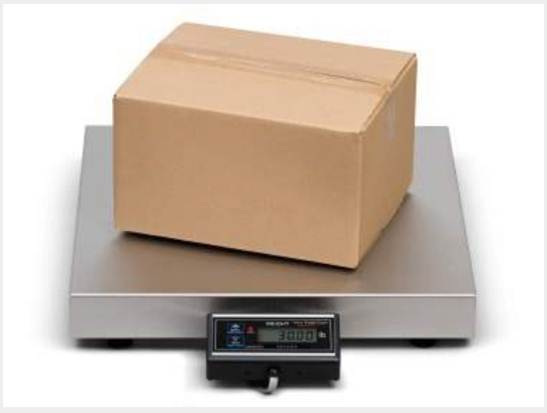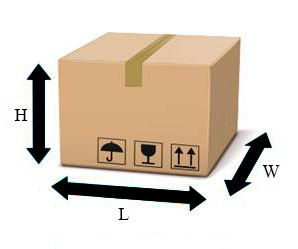### Weight Calculation

Home Weight Calculation

#### Gross Weight (Scale Weight)#### Volumetric Weight

How volumetric weight calculated? In the courier industry, also other carriers, use varying methods for assessing the volumetric weight of a cylinder, box or a parcel. Most of the courier rates base their rates on either the size or volumetric weight of the parcel, whichever is greater. The bigger packages take more room it takes up on a vehicle also in the aircraft, and the more it costs to send. If courier package is large but less in weight, the price will be based on the size rather than the scale weight.

#### Calculation of Volumetric Weight (Dimensional Weight)

Length x Width x Height in centimeters / 5000 = Volumetric Weight in kilograms rounded up to the nearest kg

### Volumetric Diagrammatic Expression

Length x Width x Height >> in cm / inches

5000 for cm / 305 for inches#### Example

• A person ships a parcel which is 1KG in actual (Scale) weight. Customers packaging Dimensions are 60cm (L) x 25cm (W) x 20cm (H)
• Multiply 60 x 25 x 20 = 15,000
• Now divide 15,000 by 5000 (Volumetric formula) to get the volumetric weight = 3KGS.
• Customer will be charged for 3KGS rather than Actual, Gross, Scale weight of 1KG.

Every shipment shall be charged by its chargeable weight. As defined here under and not the actual weight. The chargeable weight shall be higher of

• a) The actual weight rounded to the next one kilo as per the rate category agreed to or
• b) The volumetric weight similarly rounded off as mentioned in (a) above.

All your Local Pickup Requests Call Us:   +9471 422 411 5
International Pickup requests write Us:   arrowexpress@sltnet.lk